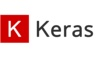keras 订阅
Keras是一个由Python编写的开源人工神经网络库，可以作为Tensorflow、Microsoft-CNTK和Theano的高阶应用程序接口，进行深度学习模型的设计、调试、评估、应用和可视化   。Keras在代码结构上由面向对象方法编写，完全模块化并具有可扩展性，其运行机制和说明文档有将用户体验和使用难度纳入考虑，并试图简化复杂算法的实现难度   。Keras支持现代人工智能领域的主流算法，包括前馈结构和递归结构的神经网络，也可以通过封装参与构建统计学习模型   。在硬件和开发环境方面，Keras支持多操作系统下的多GPU并行计算，可以根据后台设置转化为Tensorflow、Microsoft-CNTK等系统下的组件   。Keras的主要开发者是谷歌工程师François Chollet，此外其GitHub项目页面包含6名主要维护者和超过800名直接贡献者   。Keras在其正式版本公开后，除部分预编译模型外，按MIT许可证开放源代码   。 展开全文Keras是一个由Python编写的开源人工神经网络库，可以作为Tensorflow、Microsoft-CNTK和Theano的高阶应用程序接口，进行深度学习模型的设计、调试、评估、应用和可视化   。Keras在代码结构上由面向对象方法编写，完全模块化并具有可扩展性，其运行机制和说明文档有将用户体验和使用难度纳入考虑，并试图简化复杂算法的实现难度   。Keras支持现代人工智能领域的主流算法，包括前馈结构和递归结构的神经网络，也可以通过封装参与构建统计学习模型   。在硬件和开发环境方面，Keras支持多操作系统下的多GPU并行计算，可以根据后台设置转化为Tensorflow、Microsoft-CNTK等系统下的组件   。Keras的主要开发者是谷歌工程师François Chollet，此外其GitHub项目页面包含6名主要维护者和超过800名直接贡献者   。Keras在其正式版本公开后，除部分预编译模型外，按MIT许可证开放源代码   。

François Chollet 等 

Linux，macOS，Windows

0.1.0/2015年6月13日 

Keras

Python

2.3.0/2019年09月17日 
Keras历史与命名
Keras的前身是François Chollet为ONEIROS（Open-ended Neuro-Electronic Intelligent Robot Operating System）项目所的编写的代码，在2015年分离成为开源的人工神经网络工具。Keras的最初版本以Theano为后台，设计理念参考了Torch但完全由Python编写   。2015年11月的Keras测试版本0.3.0，Tensorflow被加入了后台选项。2016年4月，Keras在PIPy上发布了第一个稳定版本1.0.0   。2017年5月，Keras版本2.0.0发布   。同年6月，Keras版本2.0.5测试性地将Microsoft-CNTK加入后台选项   。自2017年起，Keras得到了Tensorflow团队的支持，其大部分组件被整合至Tensorflow的Python API中。在2018年Tensorflow 2.0.0公开后，Keras被正式确立为Tensorflow高阶API，即tf.keras   。此外自2017年7月开始，Keras也得到了CNTK 2.0的后台支持   。在2019年9月17日Keras稳定版本2.3.0的更新文档中，Keras团队宣布了计划调整：在该版本之后，Keras将集中于Tensoflow后台的有关内容，多后台Keras的开发优先度被降低，且更新将仅包含补丁（bug fix），不会有新的功能性内容移植/加入   。Keras的命名来自古希腊语“κέρας （牛角）”或 “κραίνω（实现）”，意为将梦境化为现实的“牛角之门”   。由荷马史诗《奥德赛》第19卷佩涅罗佩与奥德修斯的对话，无法挽留的梦幻拥有两座门，一座门由牛角制成，一座门由象牙制成，象牙之门内光彩夺目，却仅是无法实现的梦境；唯有走进牛角之门，才能由梦境看见真实   。

• ## keras

万次阅读 多人点赞 2016-07-31 00:15:36
Keras简介 Keras是基于Theano的一个深度学习框架，它的设计参考了Torch，用Python语言编写，是一个高度模块化的神经网络库，支持GPU和CPU。使用文档在这：http://keras.io/，这个框架貌似是刚刚火起来的，使用上的...
大神笔记，转载自http://blog.csdn.net/u012162613/article/details/45397033

Keras简介
Keras是基于Theano的一个深度学习框架，它的设计参考了Torch，用Python语言编写，是一个高度模块化的神经网络库，支持GPU和CPU。使用文档在这：http://keras.io/，这个框架貌似是刚刚火起来的，使用上的问题可以到github提issue:https://github.com/fchollet/keras

下面简单介绍一下怎么使用Keras，以Mnist数据库为例,编写一个CNN网络结构，你将会发现特别简单。

Keras里的模块介绍
Optimizers

上面的代码是SGD的使用方法，lr表示学习速率,momentum表示动量项，decay是学习速率的衰减系数(每个epoch衰减一次),Nesterov的值是False或者True，表示使不使用Nesterov momentum。其他的请参考文档。

Objectives

这是目标函数模块，keras提供了mean_squared_error，mean_absolute_error
，squared_hinge，hinge，binary_crossentropy，categorical_crossentropy这几种目标函数。

这里binary_crossentropy 和 categorical_crossentropy也就是常说的logloss.

Activations

Initializations

这是参数初始化模块，在添加layer的时候调用init进行初始化。keras提供了uniform、lecun_uniform、normal、orthogonal、zero、glorot_normal、he_normal这几种。

layers

其中core里面包含了flatten(CNN的全连接层之前需要把二维特征图flatten成为一维的)、reshape（CNN输入时将一维的向量弄成二维的）、dense(就是隐藏层，dense是稠密的意思),还有其他的就不介绍了。convolutional层基本就是Theano的Convolution2D的封装。

Preprocessing

这是预处理模块，包括序列数据的处理，文本数据的处理，图像数据的处理。重点看一下图像数据的处理，keras提供了ImageDataGenerator函数,实现data augmentation，数据集扩增，对图像做一些弹性变换，比如水平翻转，垂直翻转，旋转等。

Models

这是最主要的模块，模型。上面定义了各种基本组件，model是将它们组合起来，下面通过一个实例来说明。

3.一个实例：用CNN分类Mnist

数据下载

Mnist数据在其官网上有提供，但是不是图像格式的，因为我们通常都是直接处理图像，为了以后程序能复用，我把它弄成图像格式的，这里可以下载：http://pan.baidu.com/s/1qCdS6，共有42000张图片。

读取图片数据

keras要求输入的数据格式是numpy.array类型（numpy是一个python的数值计算的库），所以需要写一个脚本来读入mnist图像，保存为一个四维的data，还有一个一维的label，代码：

#coding:utf-8

import os
from PIL import Image
import numpy as np

#读取文件夹mnist下的42000张图片，图片为灰度图，所以为1通道，
#如果是将彩色图作为输入,则将1替换为3，并且data[i,:,:,:] = arr改为data[i,:,:,:] = [arr[:,:,0],arr[:,:,1],arr[:,:,2]]
data = np.empty((42000,1,28,28),dtype="float32")
label = np.empty((42000,),dtype="uint8")

imgs = os.listdir("./mnist")
num = len(imgs)
for i in range(num):
img = Image.open("./mnist/"+imgs[i])
arr = np.asarray(img,dtype="float32")
data[i,:,:,:] = arr
label[i] = int(imgs[i].split('.'))
return data,label

构建CNN，训练

短短二十多行代码，构建一个三个卷积层的CNN，直接读下面的代码吧，有注释，很容易读懂：

#导入各种用到的模块组件
from __future__ import absolute_import
from __future__ import print_function
from keras.preprocessing.image import ImageDataGenerator
from keras.models import Sequential
from keras.layers.core import Dense, Dropout, Activation, Flatten
from keras.layers.convolutional import Convolution2D, MaxPooling2D
from keras.utils import np_utils, generic_utils
from six.moves import range

#加载数据
print(data.shape, ' samples')

#label为0~9共10个类别，keras要求格式为binary class matrices,转化一下，直接调用keras提供的这个函数
label = np_utils.to_categorical(label, 10)

###############
#开始建立CNN模型
###############

#生成一个model
model = Sequential()

#第一个卷积层，4个卷积核，每个卷积核大小5*5。1表示输入的图片的通道,灰度图为1通道。
#border_mode可以是valid或者full，具体看这里说明：http://deeplearning.net/software/theano/library/tensor/nnet/conv.html#theano.tensor.nnet.conv.conv2d
#激活函数用tanh

#第二个卷积层，8个卷积核，每个卷积核大小3*3。4表示输入的特征图个数，等于上一层的卷积核个数
#激活函数用tanh
#采用maxpooling，poolsize为(2,2)

#第三个卷积层，16个卷积核，每个卷积核大小3*3
#激活函数用tanh
#采用maxpooling，poolsize为(2,2)

#全连接层，先将前一层输出的二维特征图flatten为一维的。
#Dense就是隐藏层。16就是上一层输出的特征图个数。4是根据每个卷积层计算出来的：(28-5+1)得到24,(24-3+1)/2得到11，(11-3+1)/2得到4
#全连接有128个神经元节点,初始化方式为normal

#Softmax分类，输出是10类别

#############
#开始训练模型
##############
#使用SGD + momentum
#model.compile里的参数loss就是损失函数(目标函数)
sgd = SGD(l2=0.0,lr=0.05, decay=1e-6, momentum=0.9, nesterov=True)
model.compile(loss='categorical_crossentropy', optimizer=sgd,class_mode="categorical")

#调用fit方法，就是一个训练过程. 训练的epoch数设为10，batch_size为100．
#数据经过随机打乱shuffle=True。verbose=1，训练过程中输出的信息，0、1、2三种方式都可以，无关紧要。show_accuracy=True，训练时每一个epoch都输出accuracy。
#validation_split=0.2，将20%的数据作为验证集。
model.fit(data, label, batch_size=100,nb_epoch=10,shuffle=True,verbose=1,show_accuracy=True,validation_split=0.2)


代码使用与结果

结果如下所示，在Epoch 9达到了0.98的训练集识别率和0.97的验证集识别率：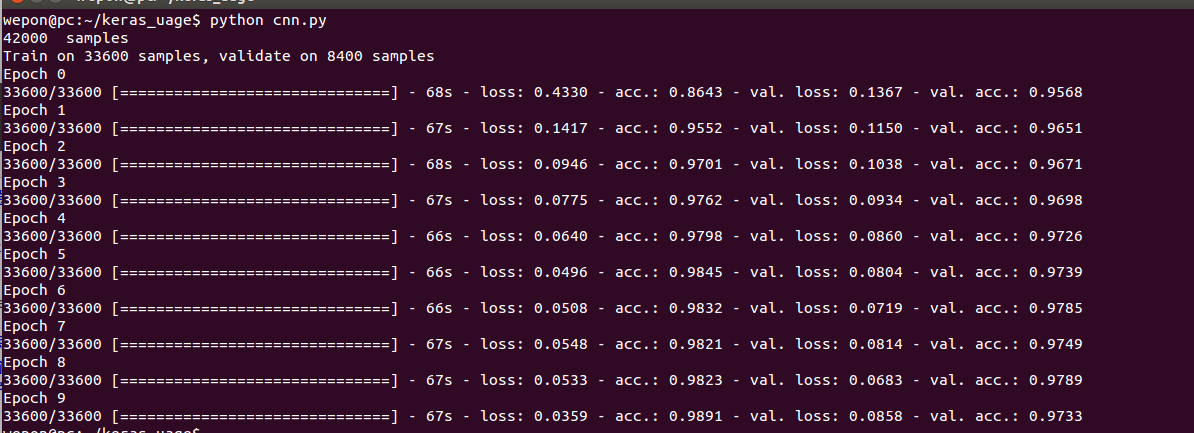展开全文• 课程内容基于最新的Keras版本（你也可以使用 TensorFlow 2 的 tf.keras 模块），其中有大量独家解读、案例，以及不少讲师一线实战多年的方法论和深度思考。同时，在层次划分上，难易兼顾，循序渐进。既有核心的基础...深度学习 深度学习框架 深度学习算法 python深度学习 深度强化学习
• ## Keras

千次阅读 2018-07-23 08:45:03
Keras是由纯python编写的基于theano/tensorflow的深度学习框架。Keras是一个高层神经网络API，支持快速实验，能够把你的idea迅速转换为结果，如果有如下需求，可以优先选择Keras： a）简易和快速的原型设计（keras...
Keras是由纯python编写的基于theano/tensorflow的深度学习框架。Keras是一个高层神经网络API，支持快速实验，能够把你的idea迅速转换为结果，如果有如下需求，可以优先选择Keras：

a）简易和快速的原型设计（keras具有高度模块化，极简，和可扩充特性）

b）支持CNN和RNN，或二者的结合

c）无缝CPU和GPU切换

Keras模块结构：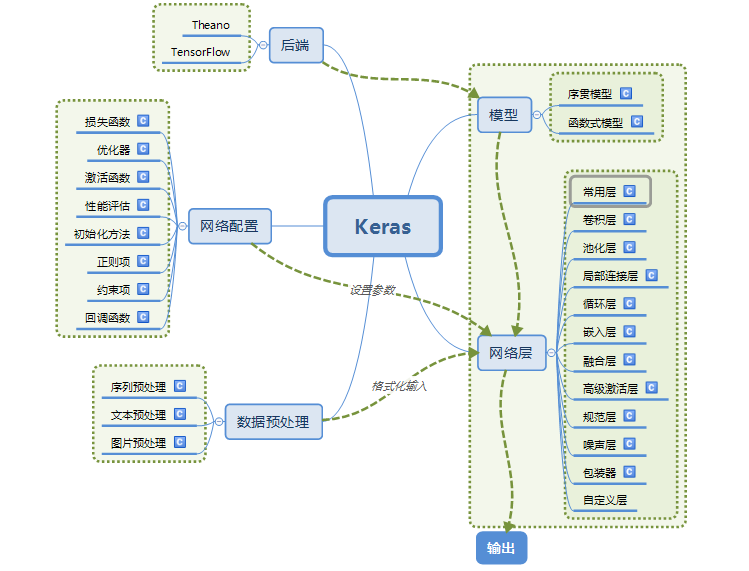使用Keras搭建一个神经网络：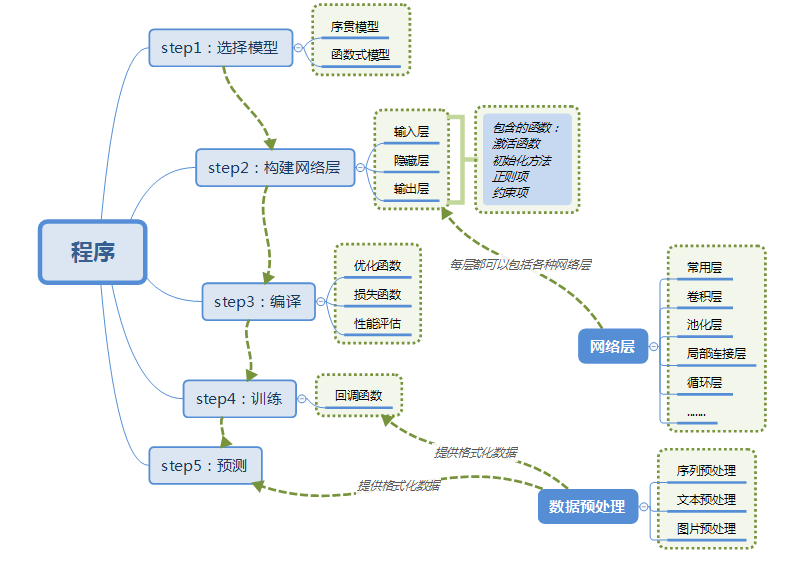【【【一些概念：

（1）目前主要有两种方式来表示张量： a) th模式或channels_first模式，Theano和caffe使用此模式。b）tf模式或channels_last模式，TensorFlow使用此模式。

例：对于100张RGB3通道的16×32（高为16宽为32）彩色图，th表示方式：（100,3,16,32）；tf表示方式：（100,16,32,3）。唯一的区别就是表示通道个数3的位置不一样。

（2）Keras有两种类型的模型，序贯模型（Sequential）和函数式模型（Model），函数式模型应用更为广泛，序贯模型是函数式模型的一种特殊情况。 a）序贯模型（Sequential）:单输入单输出，一条路通到底，层与层之间只有相邻关系，没有跨层连接。这种模型编译速度快，操作也比较简单  b）函数式模型（Model）：多输入多输出，层与层之间任意连接。这种模型编译速度慢。

（3）neural layers(神经层), cost functions(损失函数), optimizers(优化器), initialization schemes(初始化方案), activation functions(激活函数), regularization(正则化项)在keras中都是独立的模块，可以自由组合。】】】

以上内容来自：https://blog.csdn.net/zdy0_2004/article/details/74736656

一个keras model的例子（函数式模型）：

def model(input_shape):
# Define the input placeholder as a tensor with shape input_shape. Think of this as your input image!
X_input = Input(input_shape)

# CONV -> BN -> RELU Block applied to X
X = Conv2D(32, (7, 7), strides=(1, 1), name='conv0')(X)
X = BatchNormalization(axis=3, name='bn0')(X)
X = Activation('relu')(X)

# MAXPOOL
X = MaxPooling2D((2, 2), name='max_pool')(X)

# FLATTEN X (means convert it to a vector) + FULLYCONNECTED
X = Flatten()(X)
X = Dense(1, activation='sigmoid', name='fc')(X)

# Create model. This creates your Keras model instance, you'll use this instance to train/test the model.
model = Model(inputs=X_input, outputs=X, name='HappyModel')

return model


一个keras model的实例：

# number of training examples = 600
# number of test examples = 150
# X_train shape: (600, 64, 64, 3)
# Y_train shape: (600, 1)
# X_test shape: (150, 64, 64, 3)
# Y_test shape: (150, 1)
X_train_orig, Y_train_orig, X_test_orig, Y_test_orig, classes = load_dataset()

# Normalize image vectors,像素值为最大255
X_train = X_train_orig / 255.
X_test = X_test_orig / 255.

# Reshape, transfer row to column
Y_train = Y_train_orig.T
Y_test = Y_test_orig.T

def HappyModel(input_shape):
"""
Implementation of the HappyModel.

Arguments:
input_shape -- shape of the images of the dataset

Returns:
model -- a Model() instance in Keras
"""

### START CODE HERE ###
# Feel free to use the suggested outline in the text above to get started, and run through the whole
# exercise (including the later portions of this notebook) once. The come back also try out other
# network architectures as well.

# Define the input placeholder as a tensor with shape input_shape. Think of this as your input image!
X_input = Input(input_shape)

# 一种函数嵌套的形式

# CONV -> BN -> RELU Block applied to X
# 第一个参数为filters个数
X = Conv2D(32, (7, 7), strides=(1, 1), name='conv0')(X)

# axis: 整数，指定要规范化的轴，通常为特征轴。例如在进行data_format="channels_first的2D卷积后，一般会设axis=1

X = BatchNormalization(axis=3, name='bn0')(X)
X = Activation('relu')(X)

# MAXPOOL
X = MaxPooling2D((2, 2), name='max_pool')(X)

# FLATTEN X (means convert it to a vector) + FULLYCONNECTED
X = Flatten()(X)
# Dense为全连接层
X = Dense(1, activation='sigmoid', name='fc')(X)

# Create model. This creates your Keras model instance, you'll use this instance to train/test the model.
model = Model(inputs=X_input, outputs=X, name='HappyModel')

### END CODE HERE ###

return model

# mini-batch
happyModel = HappyModel(X_train.shape[1:])
happyModel.fit(x=X_train, y=Y_train, epochs=10, batch_size=32)

preds = happyModel.evaluate(X_test, Y_test)
print()
print("Loss = " + str(preds))
print("Test Accuracy = " + str(preds))

1、类Dense，

Keras.layers.core.Dense(output_dim, init='glorot_uniform', activation='linear', weights=None, W_regularizer=None, b_regularizer=None, activity_regularizer=None, W_constraint=None, b_constraint=None, input_dim=None)

ouput_dim：int > 0，输出结果的维度

init：初始化权值的函数名称或Theano function。可以使用Keras内置的，也可以传递自己编写的Theano function。如果不给weights传递参数时，则该参数必须指明。

activation：激活函数名称或者Theano function。可以使用Keras内置的，也可以是传递自己编写的Theano function。如果不明确指定，那么将没有激活函数会被应用。

weights：用于初始化权值的numpy arrays组成的list。这个List至少有1个元素，其shape为（input_dim, output_dim）。（如果指定init了，那么weights可以赋值None）2、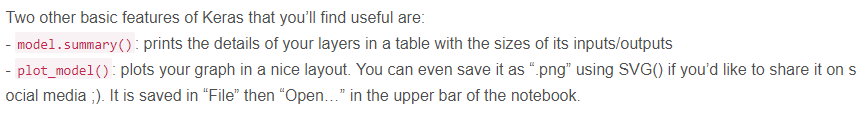3、为什么有的Keras函数后两个()？？

Dense等类都是基于Layer类的，参考class Dense(Layer)，因此我们可以看看Layer这个类怎么写的。在Layer这个类里面，有这样一个函数：def __call__(self, inputs, **kwargs)；这个函数可以在python的文档中查到，通过定义这个函数，我们可以让一个对象当作函数使用。因此我们可以把下面代码拆分成这样：

X = Dense(64, activation='relu')(X)
# 拆分
fc = Dense(64, activation='relu')
X = fc(X)

该层在每个batch上将前一层的激活值重新规范化，即使得其输出数据的均值接近0，其标准差接近1

（1）、在什么地方来进行BN：BN可以应用于网络中任意的activation set。特别指出在CNN中，BN应作用在非线性映射前，即对做规范化。

（2）、为什么用BN？克服深度神经网络难以训练的弊病，为了防止“梯度弥散”。关于梯度弥散，大家都知道一个简单的栗子：，在BN中，是通过将activation规范为均值和方差一致的手段使得原本会减小的activation的scale变大。总结起来就是BN解决了反向传播过程中的梯度问题（梯度消失和爆炸），同时使得不同scale的整体更新步调更一致

（4）、什么时候使用BN？OK，说完BN的优势，自然可以知道什么时候用BN比较好。例如，在神经网络训练时遇到收敛速度很慢，或梯度爆炸等无法训练的状况时可以尝试BN来解决。另外，在一般使用情况下也可以加入BN来加快训练速度，提高模型精度。


展开全文• Keras安装Keras是什么安装前提安装步骤安装openGV3Pycharm配置Keras Keras是什么 Keras是一个由Python编写的开源人工神经网络库，可以作为Tensorflow、Microsoft-CNTK和Theano的高阶应用程序接口，进行深度学习...
Keras安装Keras是什么安装前提安装步骤安装openGV3Pycharm配置Keras
Keras是什么

简单地说，Keras是对TensorFlow等的一个框架，方便了对TensorFlow的使用。

安装前提

在安装Keras之前需要安装TensorFlow，安装教程链接：
https://blog.csdn.net/weixin_42886817/article/details/99445098

安装步骤

在按照安装前提内的教程，安装完Anaconda和TensorFlow(CPU)之后，就会有Anaconda prompt

然后：

管理员身份打开Anaconda prompt输入activate tensorflow
conda install mingw libpython
pip install theano
pip install keras

测试Keras安装是否成功：
输入python,再输入import keras，如果没有报错，说明安装成功

安装openGV3
在上述环境下输入conda install -c menpo opencv3
Pycharm配置Keras
参考教程：

版权声明：本文为博主原创文章，遵循 CC 4.0 by-sa 版权协议，转载请附上原文出处链接和本声明。
本文链接：https://blog.csdn.net/hweiyi/article/details/70018317

在系统变量的PATH下添加：
E:\Anaconda3
E:\Anaconda3\Scripts
E:\Anaconda3\Library\bin
E:\Anaconda3\MinGW\bin
E:\Anaconda3\MinGW\x86_64-w64-mingw32\lib
E:\Anaconda3\Lib\site-packages\theano

如果 E:\Anaconda3\Lib\site-packages\下没有theano文件，可去 https://github.com/Theano/Theano 下载，将theano文件夹放到对应目录下

打开Pycharm File->Settings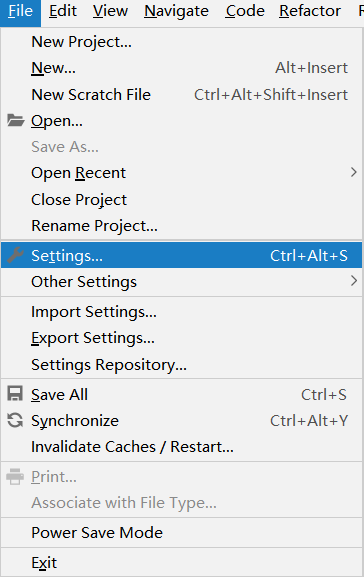Project Interpreter-Add Local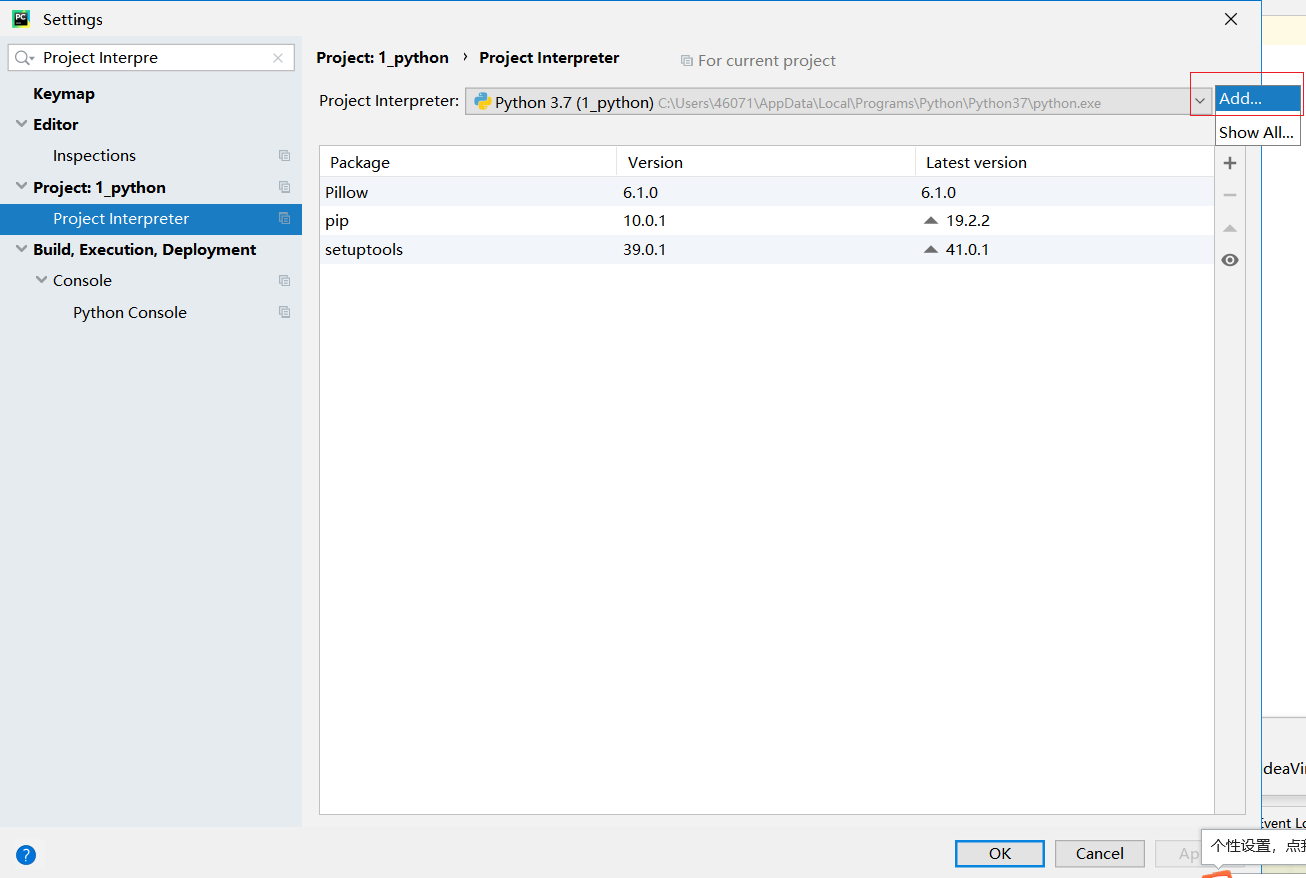选择Anaconda目录下的python.exe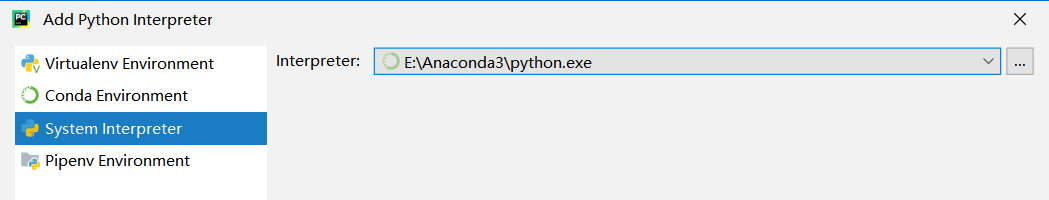展开全文• 不得不说，这深度学习框架更新太快了尤其到了Keras2.0版本，快到Keras中文版好多都是错的，快到官方文档也有旧的没更新，前路坑太多。 到发文为止，已经有theano/tensorflow/CNTK支持keras,虽然说tensorflow造势...

不得不说，这深度学习框架更新太快了尤其到了Keras2.0版本，快到Keras中文版好多都是错的，快到官方文档也有旧的没更新，前路坑太多。
到发文为止，已经有theano/tensorflow/CNTK支持keras,虽然说tensorflow造势很多，但是笔者认为接下来Keras才是正道。
笔者先学的caffe，从使用来看，比caffe简单超级多，非常好用，特别是重新训练一个模型，但是呢，在fine-tuning的时候，遇到了很多问题，对新手比较棘手。

官方文档：https://keras.io/
文档主要是以keras2.0。
.
Keras系列：
1、keras系列︱Sequential与Model模型、keras基本结构功能（一）
2、keras系列︱Application中五款已训练模型、VGG16框架（Sequential式、Model式）解读（二）
3、keras系列︱图像多分类训练与利用bottleneck features进行微调（三）
4、keras系列︱人脸表情分类与识别：opencv人脸检测+Keras情绪分类（四）
5、keras系列︱迁移学习：利用InceptionV3进行fine-tuning及预测、完整案例（五）
零、keras介绍与基本的模型保存
写成了思维导图，便于观察与理解。
1.keras网络结构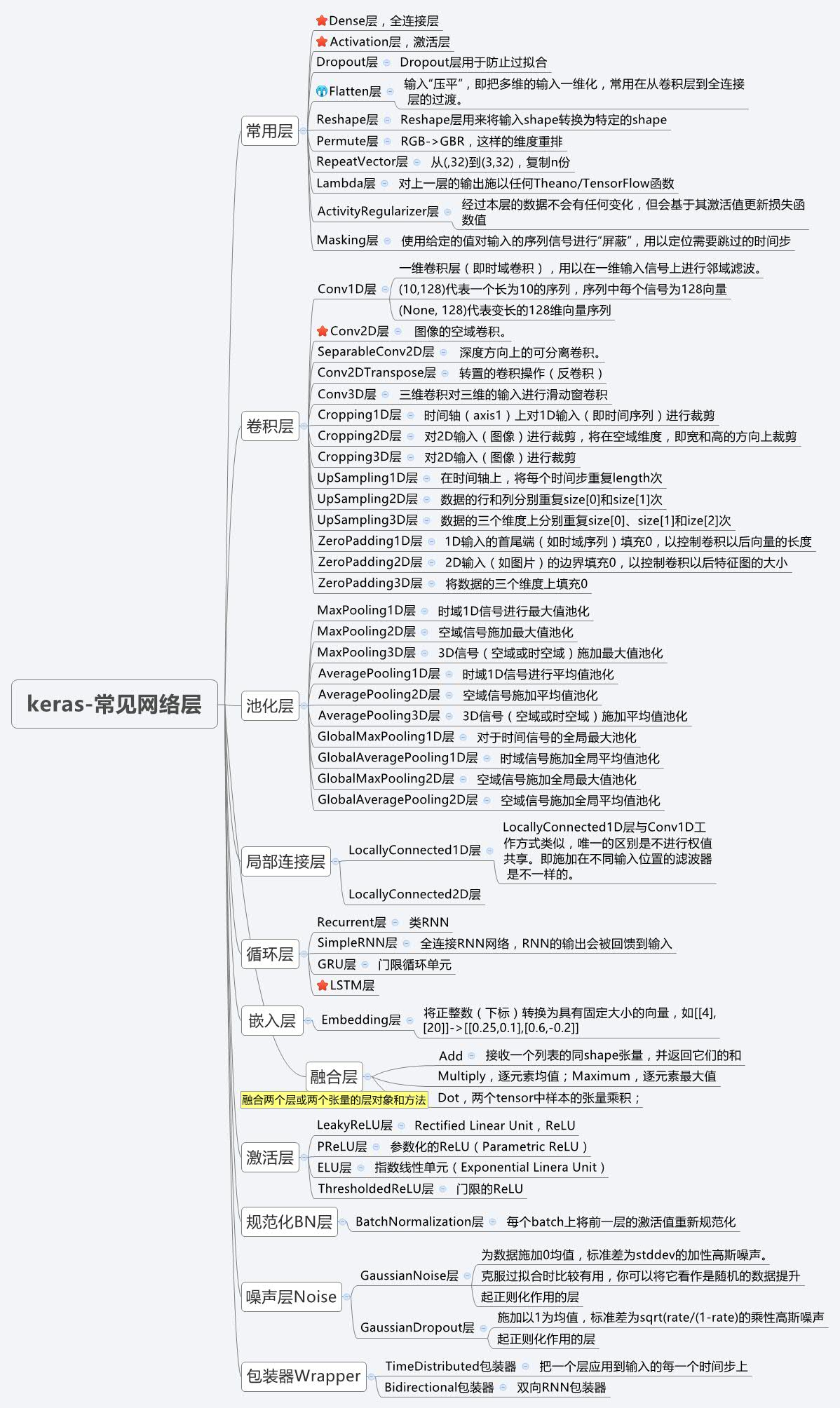###2.keras网络配置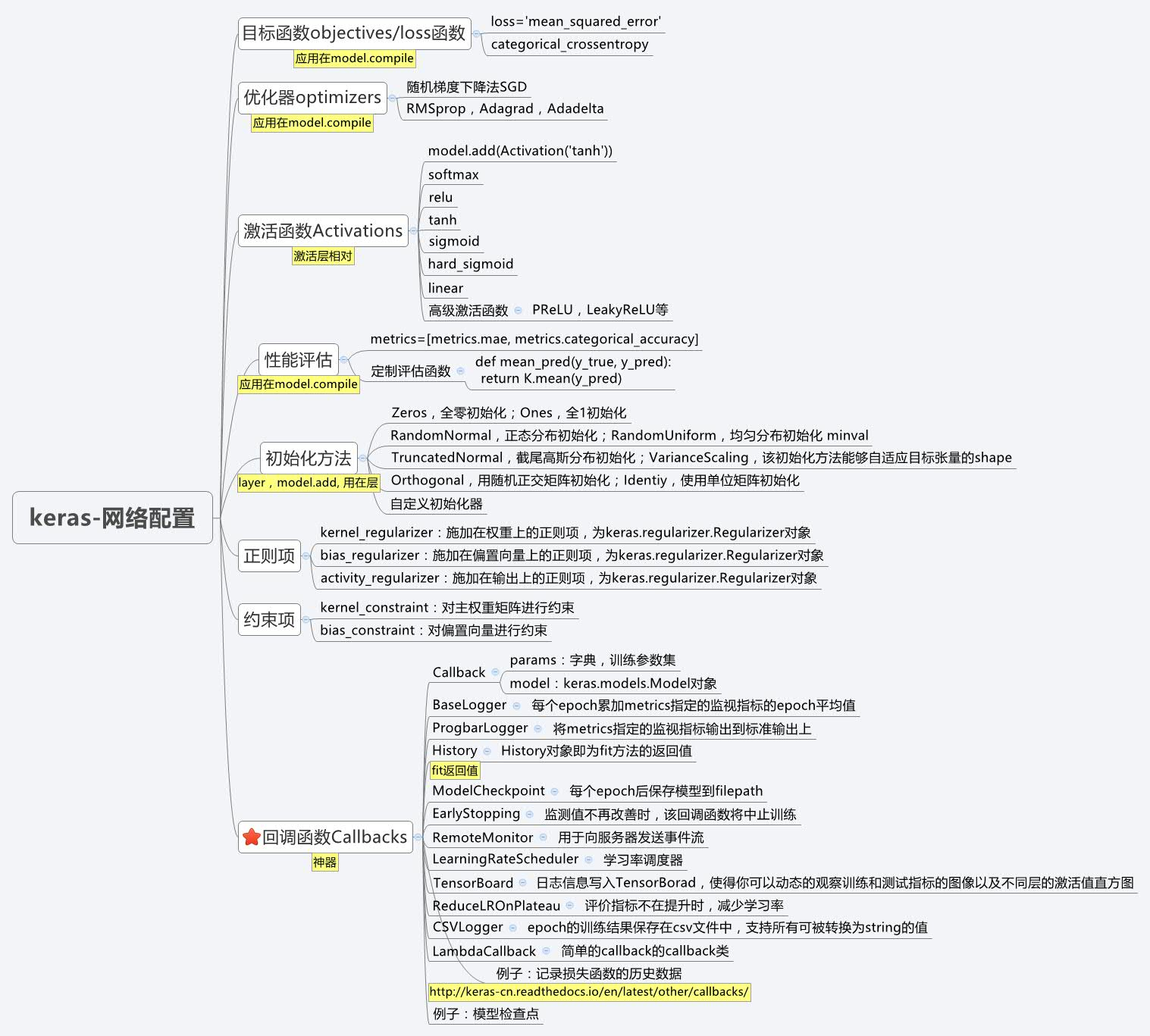其中回调函数callbacks应该是keras的精髓~
###3.keras预处理功能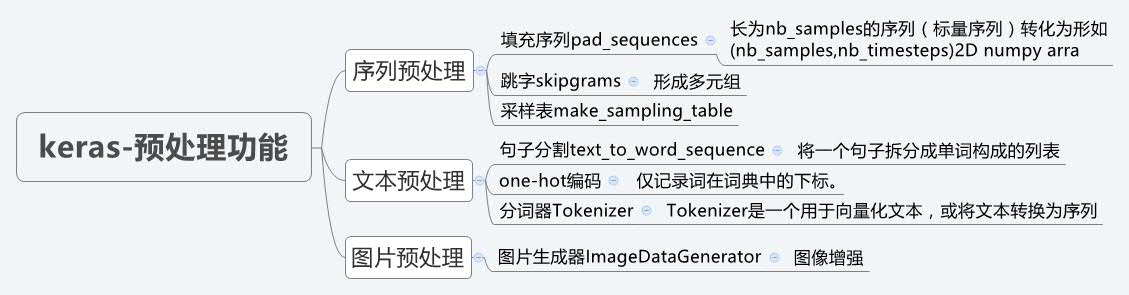###4、模型的节点信息提取
# 节点信息提取
config = model.get_config()  # 把model中的信息，solver.prototxt和train.prototxt信息提取出来
model = Model.from_config(config)  # 还回去
# or, for Sequential:
model = Sequential.from_config(config) # 重构一个新的Model模型，用去其他训练，fine-tuning比较好用

###5、 模型概况查询（包括权重查询）
# 1、模型概括打印
model.summary()

# 2、返回代表模型的JSON字符串，仅包含网络结构，不包含权值。可以从JSON字符串中重构原模型：
from models import model_from_json

json_string = model.to_json()
model = model_from_json(json_string)

# 3、model.to_yaml：与model.to_json类似，同样可以从产生的YAML字符串中重构模型
from models import model_from_yaml

yaml_string = model.to_yaml()
model = model_from_yaml(yaml_string)

# 4、权重获取
model.get_layer()      #依据层名或下标获得层对象
model.get_weights()    #返回模型权重张量的列表，类型为numpy array
model.set_weights()    #从numpy array里将权重载入给模型，要求数组具有与model.get_weights()相同的形状。

# 查看model中Layer的信息
model.layers 查看layer信息


###6、模型保存与加载
model.save_weights(filepath)
# 将模型权重保存到指定路径，文件类型是HDF5（后缀是.h5）

# 从HDF5文件中加载权重到当前模型中, 默认情况下模型的结构将保持不变。
# 如果想将权重载入不同的模型（有些层相同）中，则设置by_name=True，只有名字匹配的层才会载入权重

.
7、如何在keras中设定GPU使用的大小
本节来源于：深度学习theano/tensorflow多显卡多人使用问题集（参见：Limit the resource usage for tensorflow backend · Issue #1538 · fchollet/keras · GitHub）
在使用keras时候会出现总是占满GPU显存的情况，可以通过重设backend的GPU占用情况来进行调节。
import tensorflow as tf
from keras.backend.tensorflow_backend import set_session
config = tf.ConfigProto()
config.gpu_options.per_process_gpu_memory_fraction = 0.3
set_session(tf.Session(config=config))

需要注意的是，虽然代码或配置层面设置了对显存占用百分比阈值，但在实际运行中如果达到了这个阈值，程序有需要的话还是会突破这个阈值。换而言之如果跑在一个大数据集上还是会用到更多的显存。以上的显存限制仅仅为了在跑小数据集时避免对显存的浪费而已。（2017年2月20日补充）
8.更科学地模型训练与模型保存
filepath = 'model-ep{epoch:03d}-loss{loss:.3f}-val_loss{val_loss:.3f}.h5'
checkpoint = ModelCheckpoint(filepath, monitor='val_loss', verbose=1, save_best_only=True, mode='min')
# fit model
model.fit(x, y, epochs=20, verbose=2, callbacks=[checkpoint], validation_data=(x, y))

save_best_only打开之后，会如下：
 ETA: 3s - loss: 0.5820Epoch 00017: val_loss did not improve

如果val_loss 提高了就会保存，没有提高就不会保存。
9.如何在keras中使用tensorboard
    RUN = RUN + 1 if 'RUN' in locals() else 1   # locals() 函数会以字典类型返回当前位置的全部局部变量。

LOG_DIR = model_save_path + '/training_logs/run{}'.format(RUN)
LOG_FILE_PATH = LOG_DIR + '/checkpoint-{epoch:02d}-{val_loss:.4f}.hdf5'   # 模型Log文件以及.h5模型文件存放地址

tensorboard = TensorBoard(log_dir=LOG_DIR, write_images=True)
checkpoint = ModelCheckpoint(filepath=LOG_FILE_PATH, monitor='val_loss', verbose=1, save_best_only=True)
early_stopping = EarlyStopping(monitor='val_loss', patience=5, verbose=1)

history = model.fit_generator(generator=gen.generate(True), steps_per_epoch=int(gen.train_batches / 4),
validation_data=gen.generate(False), validation_steps=int(gen.val_batches / 4),
epochs=EPOCHS, verbose=1, callbacks=[tensorboard, checkpoint, early_stopping])

都是在回调函数中起作用：

EarlyStopping patience：当early
（1）stop被激活（如发现loss相比上一个epoch训练没有下降），则经过patience个epoch后停止训练。
（2）mode：‘auto’，‘min’，‘max’之一，在min模式下，如果检测值停止下降则中止训练。在max模式下，当检测值不再上升则停止训练。

模型检查点ModelCheckpoint
（1）save_best_only：当设置为True时，将只保存在验证集上性能最好的模型
（2） mode：‘auto’，‘min’，‘max’之一，在save_best_only=True时决定性能最佳模型的评判准则，例如，当监测值为val_acc时，模式应为max，当检测值为val_loss时，模式应为min。在auto模式下，评价准则由被监测值的名字自动推断。
（3）save_weights_only：若设置为True，则只保存模型权重，否则将保存整个模型（包括模型结构，配置信息等）
（4）period：CheckPoint之间的间隔的epoch数

可视化tensorboard write_images: 是否将模型权重以图片的形式可视化

其他内容可参考keras中文文档
.
一、Sequential 序贯模型
序贯模型是函数式模型的简略版，为最简单的线性、从头到尾的结构顺序，不分叉。
Sequential模型的基本组件
一般需要：
2、model.compile,模型训练的BP模式设置；
3、model.fit，模型训练参数设置 + 训练；
4、模型评估
5、模型预测
add(self, layer)

# 譬如：

2、compile 训练模式——solver.prototxt文件
compile(self, optimizer, loss, metrics=None, sample_weight_mode=None)

其中：
optimizer：   字符串（预定义优化器名）或优化器对象，参考优化器
loss：        字符串（预定义损失函数名）或目标函数，参考损失函数
metrics：     列表，包含评估模型在训练和测试时的网络性能的指标，典型用法是metrics=[‘accuracy’]
sample_weight_mode：如果你需要按时间步为样本赋权（2D权矩阵），将该值设为“temporal”。
默认为“None”，代表按样本赋权（1D权）。在下面fit函数的解释中有相关的参考内容。
kwargs：       使用TensorFlow作为后端请忽略该参数，若使用Theano作为后端，kwargs的值将会传递给 K.function
注意：
模型在使用前必须编译，否则在调用fit或evaluate时会抛出异常。
3、fit 模型训练参数+训练——train.sh+soler.prototxt（部分）
fit(self, x, y, batch_size=32, epochs=10, verbose=1, callbacks=None, validation_split=0.0, validation_data=None, shuffle=True, class_weight=None, sample_weight=None, initial_epoch=0)

本函数将模型训练nb_epoch轮，其参数有：
x：输入数据。如果模型只有一个输入，那么x的类型是numpy
array，如果模型有多个输入，那么x的类型应当为list，list的元素是对应于各个输入的numpy array
y：标签，numpy array
batch_size：整数，指定进行梯度下降时每个batch包含的样本数。训练时一个batch的样本会被计算一次梯度下降，使目标函数优化一步。
epochs：整数，训练的轮数，每个epoch会把训练集轮一遍。
verbose：日志显示，0为不在标准输出流输出日志信息，1为输出进度条记录，2为每个epoch输出一行记录
callbacks：list，其中的元素是keras.callbacks.Callback的对象。这个list中的回调函数将会在训练过程中的适当时机被调用，参考回调函数
validation_split：0~1之间的浮点数，用来指定训练集的一定比例数据作为验证集。验证集将不参与训练，并在每个epoch结束后测试的模型的指标，如损失函数、精确度等。注意，validation_split的划分在shuffle之前，因此如果你的数据本身是有序的，需要先手工打乱再指定validation_split，否则可能会出现验证集样本不均匀。
validation_data：形式为（X，y）的tuple，是指定的验证集。此参数将覆盖validation_spilt。
shuffle：布尔值或字符串，一般为布尔值，表示是否在训练过程中随机打乱输入样本的顺序。若为字符串“batch”，则是用来处理HDF5数据的特殊情况，它将在batch内部将数据打乱。
class_weight：字典，将不同的类别映射为不同的权值，该参数用来在训练过程中调整损失函数（只能用于训练）
sample_weight：权值的numpy
array，用于在训练时调整损失函数（仅用于训练）。可以传递一个1D的与样本等长的向量用于对样本进行1对1的加权，或者在面对时序数据时，传递一个的形式为（samples，sequence_length）的矩阵来为每个时间步上的样本赋不同的权。这种情况下请确定在编译模型时添加了sample_weight_mode=‘temporal’。
initial_epoch: 从该参数指定的epoch开始训练，在继续之前的训练时有用。
fit函数返回一个History的对象，其History.history属性记录了损失函数和其他指标的数值随epoch变化的情况，如果有验证集的话，也包含了验证集的这些指标变化情况注意：
要与之后的fit_generator做区别，两者输入x/y不同。
4.evaluate 模型评估
evaluate(self, x, y, batch_size=32, verbose=1, sample_weight=None)

本函数按batch计算在某些输入数据上模型的误差，其参数有：
x：输入数据，与fit一样，是numpy array或numpy array的list
y：标签，numpy array
batch_size：整数，含义同fit的同名参数
verbose：含义同fit的同名参数，但只能取0或1
sample_weight：numpy array，含义同fit的同名参数
本函数返回一个测试误差的标量值（如果模型没有其他评价指标），或一个标量的list（如果模型还有其他的评价指标）。model.metrics_names将给出list中各个值的含义。
如果没有特殊说明，以下函数的参数均保持与fit的同名参数相同的含义
如果没有特殊说明，以下函数的verbose参数（如果有）均只能取0或1
5 predict 模型评估
predict(self, x, batch_size=32, verbose=0)
predict_classes(self, x, batch_size=32, verbose=1)
predict_proba(self, x, batch_size=32, verbose=1)

本函数按batch获得输入数据对应的输出，其参数有：
函数的返回值是预测值的numpy array
predict_classes：本函数按batch产生输入数据的类别预测结果；
predict_proba：本函数按batch产生输入数据属于各个类别的概率
6 on_batch 、batch的结果，检查
train_on_batch(self, x, y, class_weight=None, sample_weight=None)
test_on_batch(self, x, y, sample_weight=None)
predict_on_batch(self, x)

train_on_batch：本函数在一个batch的数据上进行一次参数更新，函数返回训练误差的标量值或标量值的list，与evaluate的情形相同。
test_on_batch：本函数在一个batch的样本上对模型进行评估，函数的返回与evaluate的情形相同
predict_on_batch：本函数在一个batch的样本上对模型进行测试，函数返回模型在一个batch上的预测结果
7 fit_generator
#利用Python的生成器，逐个生成数据的batch并进行训练。
#生成器与模型将并行执行以提高效率。
#例如，该函数允许我们在CPU上进行实时的数据提升，同时在GPU上进行模型训练

有了该函数，图像分类训练任务变得很简单。
fit_generator(self, generator, steps_per_epoch, epochs=1, verbose=1, callbacks=None, validation_data=None, validation_steps=None, class_weight=None, max_q_size=10, workers=1, pickle_safe=False, initial_epoch=0)

# 案例：
def generate_arrays_from_file(path):
while 1:
f = open(path)
for line in f:
# create Numpy arrays of input data
# and labels, from each line in the file
x, y = process_line(line)
yield (x, y)
f.close()

model.fit_generator(generate_arrays_from_file('/my_file.txt'),
samples_per_epoch=10000, epochs=10)

其他的两个辅助的内容：
evaluate_generator(self, generator, steps, max_q_size=10, workers=1, pickle_safe=False)
predict_generator(self, generator, steps, max_q_size=10, workers=1, pickle_safe=False, verbose=0)

evaluate_generator：本函数使用一个生成器作为数据源评估模型，生成器应返回与test_on_batch的输入数据相同类型的数据。该函数的参数与fit_generator同名参数含义相同，steps是生成器要返回数据的轮数。
predcit_generator：本函数使用一个生成器作为数据源预测模型，生成器应返回与test_on_batch的输入数据相同类型的数据。该函数的参数与fit_generator同名参数含义相同，steps是生成器要返回数据的轮数。
案例一：简单的2分类
For a single-input model with 2 classes (binary classification):
from keras.models import Sequential
from keras.layers import Dense, Activation

#模型搭建阶段
model= Sequential()
# Dense(32) is a fully-connected layer with 32 hidden units.
model.compile(optimizer='rmsprop',
loss='binary_crossentropy',
metrics=['accuracy'])

其中：
Sequential()代表类的初始化；
Dense代表全连接层，此时有32个神经元，最后接relu，输入的是100维度
compile，跟prototxt一样，一些训练参数,solver.prototxt
# Generate dummy data
import numpy as np
data = np.random.random((1000, 100))
labels = np.random.randint(2, size=(1000, 1))

# Train the model, iterating on the data in batches of 32 samples
model.fit(data, labels, nb_epoch =10, batch_size=32)

之前报过这样的错误，是因为版本的问题。 版本1.2里面是nb_epoch ，而keras2.0是epochs = 10
 error:
TypeError: Received unknown keyword arguments: {'epochs': 10}

其中：
epoch=batch_size * iteration,10次epoch代表训练十次训练集
案例二:多分类-VGG的卷积神经网络
import numpy as np
import keras
from keras.models import Sequential
from keras.layers import Dense, Dropout, Flatten
from keras.layers import Conv2D, MaxPooling2D
from keras.optimizers import SGD
from keras.utils import np_utils

# Generate dummy data
x_train = np.random.random((100, 100, 100, 3))
# 100张图片，每张100*100*3
y_train = keras.utils.to_categorical(np.random.randint(10, size=(100, 1)), num_classes=10)
# 100*10
x_test = np.random.random((20, 100, 100, 3))
y_test = keras.utils.to_categorical(np.random.randint(10, size=(20, 1)), num_classes=10)
# 20*100

model = Sequential()
# input: 100x100 images with 3 channels -> (100, 100, 3) tensors.
# this applies 32 convolution filters of size 3x3 each.
model.add(Conv2D(32, (3, 3), activation='relu', input_shape=(100, 100, 3)))

sgd = SGD(lr=0.01, decay=1e-6, momentum=0.9, nesterov=True)
model.compile(loss='categorical_crossentropy', optimizer=sgd)

model.fit(x_train, y_train, batch_size=32, epochs=10)
score = model.evaluate(x_test, y_test, batch_size=32)

标准序贯网络，标签的训练模式注意：
这里非常重要的一点，对于我这样的新手，这一步的作用？
keras.utils.to_categorical

特别是多分类时候，我之前以为输入的就是一列（100，），但是keras在多分类任务中是不认得这个的，所以需要再加上这一步，让其转化为Keras认得的数据格式。
案例三：使用LSTM的序列分类
from keras.models import Sequential
from keras.layers import Dense, Dropout
from keras.layers import Embedding
from keras.layers import LSTM

model = Sequential()

model.compile(loss='binary_crossentropy',
optimizer='rmsprop',
metrics=['accuracy'])

model.fit(x_train, y_train, batch_size=16, epochs=10)
score = model.evaluate(x_test, y_test, batch_size=16)

.
三、Model式模型
比序贯模型要复杂，但是效果很好，可以同时/分阶段输入变量，分阶段输出想要的模型；
一句话，只要你的模型不是类似VGG一样一条路走到黑的模型，或者你的模型需要多于一个的输出，那么你总应该选择函数式模型。
不同之处：
书写结构完全不一致
函数式模型基本属性与训练流程
一般需要：
1、model.layers，添加层信息；
2、model.compile,模型训练的BP模式设置；
3、model.fit，模型训练参数设置 + 训练；
4、evaluate，模型评估；
5、predict 模型预测
1 常用Model属性
model.layers：组成模型图的各个层
model.inputs：模型的输入张量列表
model.outputs：模型的输出张量列表

2 compile 训练模式设置——solver.prototxt
compile(self, optimizer, loss, metrics=None, loss_weights=None, sample_weight_mode=None)

本函数编译模型以供训练，参数有
optimizer：优化器，为预定义优化器名或优化器对象，参考优化器
loss：损失函数，为预定义损失函数名或一个目标函数，参考损失函数
metrics：列表，包含评估模型在训练和测试时的性能的指标，典型用法是metrics=[‘accuracy’]如果要在多输出模型中为不同的输出指定不同的指标，可像该参数传递一个字典，例如metrics={‘ouput_a’: ‘accuracy’}
sample_weight_mode：如果你需要按时间步为样本赋权（2D权矩阵），将该值设为“temporal”。默认为“None”，代表按样本赋权（1D权）。
如果模型有多个输出，可以向该参数传入指定sample_weight_mode的字典或列表。在下面fit函数的解释中有相关的参考内容。
【Tips】如果你只是载入模型并利用其predict，可以不用进行compile。在Keras中，compile主要完成损失函数和优化器的一些配置，是为训练服务的。predict会在内部进行符号函数的编译工作（通过调用_make_predict_function生成函数）
3  fit 模型训练参数设置 + 训练
fit(self, x=None, y=None, batch_size=32, epochs=1, verbose=1, callbacks=None, validation_split=0.0, validation_data=None, shuffle=True, class_weight=None, sample_weight=None, initial_epoch=0)

本函数用以训练模型，参数有：
x：输入数据。如果模型只有一个输入，那么x的类型是numpy
array，如果模型有多个输入，那么x的类型应当为list，list的元素是对应于各个输入的numpy
array。如果模型的每个输入都有名字，则可以传入一个字典，将输入名与其输入数据对应起来。
y：标签，numpy array。如果模型有多个输出，可以传入一个numpy
array的list。如果模型的输出拥有名字，则可以传入一个字典，将输出名与其标签对应起来。
batch_size：整数，指定进行梯度下降时每个batch包含的样本数。训练时一个batch的样本会被计算一次梯度下降，使目标函数优化一步。
nb_epoch：整数，训练的轮数，训练数据将会被遍历nb_epoch次。Keras中nb开头的变量均为"number of"的意思
verbose：日志显示，0为不在标准输出流输出日志信息，1为输出进度条记录，2为每个epoch输出一行记录
callbacks：list，其中的元素是keras.callbacks.Callback的对象。这个list中的回调函数将会在训练过程中的适当时机被调用，参考回调函数
validation_split：0~1之间的浮点数，用来指定训练集的一定比例数据作为验证集。验证集将不参与训练，并在每个epoch结束后测试的模型的指标，如损失函数、精确度等。注意，validation_split的划分在shuffle之后，因此如果你的数据本身是有序的，需要先手工打乱再指定validation_split，否则可能会出现验证集样本不均匀。
validation_data：形式为（X，y）或（X，y，sample_weights）的tuple，是指定的验证集。此参数将覆盖validation_spilt。
shuffle：布尔值，表示是否在训练过程中每个epoch前随机打乱输入样本的顺序。
class_weight：字典，将不同的类别映射为不同的权值，该参数用来在训练过程中调整损失函数（只能用于训练）。该参数在处理非平衡的训练数据（某些类的训练样本数很少）时，可以使得损失函数对样本数不足的数据更加关注。
sample_weight：权值的numpy
array，用于在训练时调整损失函数（仅用于训练）。可以传递一个1D的与样本等长的向量用于对样本进行1对1的加权，或者在面对时序数据时，传递一个的形式为（samples，sequence_length）的矩阵来为每个时间步上的样本赋不同的权。这种情况下请确定在编译模型时添加了sample_weight_mode=‘temporal’。
initial_epoch: 从该参数指定的epoch开始训练，在继续之前的训练时有用。
输入数据与规定数据不匹配时会抛出错误
fit函数返回一个History的对象，其History.history属性记录了损失函数和其他指标的数值随epoch变化的情况，如果有验证集的话，也包含了验证集的这些指标变化情况
4.evaluate，模型评估
evaluate(self, x, y, batch_size=32, verbose=1, sample_weight=None)

本函数按batch计算在某些输入数据上模型的误差，其参数有：
x：输入数据，与fit一样，是numpy array或numpy array的list
y：标签，numpy array
batch_size：整数，含义同fit的同名参数
verbose：含义同fit的同名参数，但只能取0或1
sample_weight：numpy array，含义同fit的同名参数
本函数返回一个测试误差的标量值（如果模型没有其他评价指标），或一个标量的list（如果模型还有其他的评价指标）。model.metrics_names将给出list中各个值的含义。
如果没有特殊说明，以下函数的参数均保持与fit的同名参数相同的含义
如果没有特殊说明，以下函数的verbose参数（如果有）均只能取0或1
5.predict 模型预测
predict(self, x, batch_size=32, verbose=0)


本函数按batch获得输入数据对应的输出，其参数有：
函数的返回值是预测值的numpy array
模型检查 on_batch
train_on_batch(self, x, y, class_weight=None, sample_weight=None)
test_on_batch(self, x, y, sample_weight=None)
predict_on_batch(self, x)

train_on_batch：本函数在一个batch的数据上进行一次参数更新，函数返回训练误差的标量值或标量值的list，与evaluate的情形相同。
test_on_batch：本函数在一个batch的样本上对模型进行评估，函数的返回与evaluate的情形相同；
predict_on_batch：本函数在一个batch的样本上对模型进行测试，函数返回模型在一个batch上的预测结果
_generator
fit_generator(self, generator, steps_per_epoch, epochs=1, verbose=1, callbacks=None, validation_data=None, validation_steps=None, class_weight=None, max_q_size=10, workers=1, pickle_safe=False, initial_epoch=0)
evaluate_generator(self, generator, steps, max_q_size=10, workers=1, pickle_safe=False)

案例一：简单的单层-全连接网络
from keras.layers import Input, Dense
from keras.models import Model

# This returns a tensor
inputs = Input(shape=(784,))

# a layer instance is callable on a tensor, and returns a tensor
x = Dense(64, activation='relu')(inputs)
# 输入inputs，输出x
# (inputs)代表输入
x = Dense(64, activation='relu')(x)
# 输入x，输出x
predictions = Dense(10, activation='softmax')(x)
# 输入x，输出分类

# This creates a model that includes
# the Input layer and three Dense layers
model = Model(inputs=inputs, outputs=predictions)
model.compile(optimizer='rmsprop',
loss='categorical_crossentropy',
metrics=['accuracy'])
model.fit(data, labels)  # starts training

其中：
可以看到结构与序贯模型完全不一样，其中x = Dense(64, activation=‘relu’)(inputs)中：(input)代表输入；x代表输出
model = Model(inputs=inputs, outputs=predictions)；该句是函数式模型的经典，可以同时输入两个input，然后输出output两个模型
案例二：视频处理
x = Input(shape=(784,))
# This works, and returns the 10-way softmax we defined above.
y = model(x)
# model里面存着权重，然后输入x，输出结果，用来作fine-tuning

# 分类->视频、实时处理
from keras.layers import TimeDistributed

# Input tensor for sequences of 20 timesteps,
# each containing a 784-dimensional vector
input_sequences = Input(shape=(20, 784))
# 20个时间间隔，输入784维度的数据

# This applies our previous model to every timestep in the input sequences.
# the output of the previous model was a 10-way softmax,
# so the output of the layer below will be a sequence of 20 vectors of size 10.
processed_sequences = TimeDistributed(model)(input_sequences)
# Model是已经训练好的

其中：
Model是已经训练好的，现在用来做迁移学习；
其中还可以通过TimeDistributed来进行实时预测；
TimeDistributed(model)(input_sequences)，input_sequences代表序列输入；model代表已训练的模型
案例三：双输入、双模型输出：LSTM 时序预测
本案例很好，可以了解到Model的精髓在于他的任意性，给编译者很多的便利。
输入：
新闻语料；新闻语料对应的时间
输出：
新闻语料的预测模型；新闻语料+对应时间的预测模型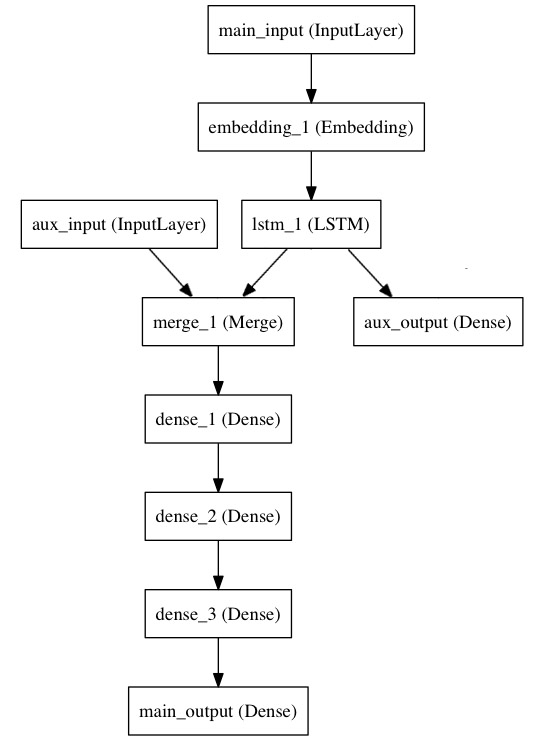模型一：只针对新闻语料的LSTM模型
from keras.layers import Input, Embedding, LSTM, Dense
from keras.models import Model

# Headline input: meant to receive sequences of 100 integers, between 1 and 10000.
# Note that we can name any layer by passing it a "name" argument.
main_input = Input(shape=(100,), dtype='int32', name='main_input')
# 一个100词的BOW序列

# This embedding layer will encode the input sequence
# into a sequence of dense 512-dimensional vectors.
x = Embedding(output_dim=512, input_dim=10000, input_length=100)(main_input)
# Embedding层，把100维度再encode成512的句向量，10000指的是词典单词总数

# A LSTM will transform the vector sequence into a single vector,
# containing information about the entire sequence
lstm_out = LSTM(32)(x)
# ？ 32什么意思？？？？？？？？？？？？？？？？？？？？？

#然后，我们插入一个额外的损失，使得即使在主损失很高的情况下，LSTM和Embedding层也可以平滑的训练。

auxiliary_output = Dense(1, activation='sigmoid', name='aux_output')(lstm_out)
#再然后，我们将LSTM与额外的输入数据串联起来组成输入，送入模型中：
# 模型一：只针对以上的序列做的预测模型

组合模型：新闻语料+时序
# 模型二：组合模型
auxiliary_input = Input(shape=(5,), name='aux_input')  # 新加入的一个Input,5维度
x = keras.layers.concatenate([lstm_out, auxiliary_input])   # 组合起来，对应起来

# We stack a deep densely-connected network on top
# 组合模型的形式
x = Dense(64, activation='relu')(x)
x = Dense(64, activation='relu')(x)
x = Dense(64, activation='relu')(x)
# And finally we add the main logistic regression layer
main_output = Dense(1, activation='sigmoid', name='main_output')(x)

#最后，我们定义整个2输入，2输出的模型：
model = Model(inputs=[main_input, auxiliary_input], outputs=[main_output, auxiliary_output])
#模型定义完毕，下一步编译模型。
#我们给额外的损失赋0.2的权重。我们可以通过关键字参数loss_weights或loss来为不同的输出设置不同的损失函数或权值。
#这两个参数均可为Python的列表或字典。这里我们给loss传递单个损失函数，这个损失函数会被应用于所有输出上。


其中：Model(inputs=[main_input, auxiliary_input], outputs=[main_output, auxiliary_output])是核心，
Input两个内容，outputs两个模型
# 训练方式一：两个模型一个loss
model.compile(optimizer='rmsprop', loss='binary_crossentropy',
loss_weights=[1., 0.2])
#编译完成后，我们通过传递训练数据和目标值训练该模型：

epochs=50, batch_size=32)

# 训练方式二：两个模型,两个Loss
#因为我们输入和输出是被命名过的（在定义时传递了“name”参数），我们也可以用下面的方式编译和训练模型：
model.compile(optimizer='rmsprop',
loss={'main_output': 'binary_crossentropy', 'aux_output': 'binary_crossentropy'},
loss_weights={'main_output': 1., 'aux_output': 0.2})

# And trained it via:
{'main_output': labels, 'aux_output': labels},
epochs=50, batch_size=32)


因为输入两个，输出两个模型，所以可以分为设置不同的模型训练参数
案例四：共享层：对应关系、相似性
一个节点，分成两个分支出去
import keras
from keras.layers import Input, LSTM, Dense
from keras.models import Model

tweet_a = Input(shape=(140, 256))
tweet_b = Input(shape=(140, 256))
#若要对不同的输入共享同一层，就初始化该层一次，然后多次调用它
# 140个单词，每个单词256维度，词向量
#

# This layer can take as input a matrix
# and will return a vector of size 64
shared_lstm = LSTM(64)
# 返回一个64规模的向量

# When we reuse the same layer instance
# multiple times, the weights of the layer
# are also being reused
# (it is effectively *the same* layer)
encoded_a = shared_lstm(tweet_a)
encoded_b = shared_lstm(tweet_b)

# We can then concatenate the two vectors:
# 连接两个结果
# axis=-1？？？？？
merged_vector = keras.layers.concatenate([encoded_a, encoded_b], axis=-1)

# And add a logistic regression on top
predictions = Dense(1, activation='sigmoid')(merged_vector)
# 其中的1 代表什么？？？？

# We define a trainable model linking the
# tweet inputs to the predictions
model = Model(inputs=[tweet_a, tweet_b], outputs=predictions)

model.compile(optimizer='rmsprop',
loss='binary_crossentropy',
metrics=['accuracy'])
model.fit([data_a, data_b], labels, epochs=10)
# 训练模型，然后预测

案例五：抽取层节点内容
# 1、单节点
a = Input(shape=(140, 256))
lstm = LSTM(32)
encoded_a = lstm(a)
assert lstm.output == encoded_a
# 抽取获得encoded_a的输出张量

# 2、多节点
a = Input(shape=(140, 256))
b = Input(shape=(140, 256))

lstm = LSTM(32)
encoded_a = lstm(a)
encoded_b = lstm(b)

assert lstm.get_output_at(0) == encoded_a
assert lstm.get_output_at(1) == encoded_b

# 3、图像层节点
# 对于input_shape和output_shape也是一样，如果一个层只有一个节点，
#或所有的节点都有相同的输入或输出shape，
#那么input_shape和output_shape都是没有歧义的，并也只返回一个值。
#但是，例如你把一个相同的Conv2D应用于一个大小为(3,32,32)的数据，
#然后又将其应用于一个(3,64,64)的数据，那么此时该层就具有了多个输入和输出的shape，
#你就需要显式的指定节点的下标，来表明你想取的是哪个了
a = Input(shape=(3, 32, 32))
b = Input(shape=(3, 64, 64))

conv = Conv2D(16, (3, 3), padding='same')
conved_a = conv(a)

# Only one input so far, the following will work:
assert conv.input_shape == (None, 3, 32, 32)

conved_b = conv(b)
# now the .input_shape property wouldn't work, but this does:
assert conv.get_input_shape_at(0) == (None, 3, 32, 32)
assert conv.get_input_shape_at(1) == (None, 3, 64, 64)

案例六：视觉问答模型
#这个模型将自然语言的问题和图片分别映射为特征向量，
#将二者合并后训练一个logistic回归层，从一系列可能的回答中挑选一个。
from keras.layers import Conv2D, MaxPooling2D, Flatten
from keras.layers import Input, LSTM, Embedding, Dense
from keras.models import Model, Sequential

# First, let's define a vision model using a Sequential model.
# This model will encode an image into a vector.
vision_model = Sequential()

# Now let's get a tensor with the output of our vision model:
image_input = Input(shape=(3, 224, 224))
encoded_image = vision_model(image_input)

# Next, let's define a language model to encode the question into a vector.
# Each question will be at most 100 word long,
# and we will index words as integers from 1 to 9999.
question_input = Input(shape=(100,), dtype='int32')
embedded_question = Embedding(input_dim=10000, output_dim=256, input_length=100)(question_input)
encoded_question = LSTM(256)(embedded_question)

# Let's concatenate the question vector and the image vector:
merged = keras.layers.concatenate([encoded_question, encoded_image])

# And let's train a logistic regression over 1000 words on top:
output = Dense(1000, activation='softmax')(merged)

# This is our final model:
vqa_model = Model(inputs=[image_input, question_input], outputs=output)

# The next stage would be training this model on actual data.

.
延伸一：fine-tuning时如何加载No_top的权重
如果你需要加载权重到不同的网络结构（有些层一样）中，例如fine-tune或transfer-learning，你可以通过层名字来加载模型：
例如：
假如原模型为：
    model = Sequential()
...
model.save_weights(fname)

# new model
model = Sequential()

# load weights from first model; will only affect the first layer, dense_1.

延伸二：应对不均衡样本的情况
使用：class_weight,sample_weight
两者的区别为：

class_weight—主要针对的上数据不均衡问题，比如：异常检测的二项分类问题，异常数据仅占1%，正常数据占99%;   此时就要设置不同类对loss的影响。

sample_weight—主要解决的是样本质量不同的问题，比如前1000个样本的可信度，那么它的权重就要高，后1000个样本可能有错、不可信，那么权重就要调低。

class_weight的使用：
cw = {0: 1, 1: 50}
model.fit(x_train, y_train,batch_size=batch_size,epochs=epochs,verbose=1,callbacks=cbks,validation_data=(x_test, y_test), shuffle=True,class_weight=cw)

sample_weight的使用：
来源：https://www.kaggle.com/c/jigsaw-toxic-comment-classification-challenge/discussion/46673
from sklearn.utils import class_weight

list_classes = ["toxic", "severe_toxic", "obscene", "threat", "insult", "identity_hate"]
y = train[list_classes].values
sample_weights = class_weight.compute_sample_weight('balanced', y)

model.fit(X_t, y, batch_size=batch_size, epochs=epochs,validation_split=0.1,sample_weight=sample_weights, callbacks=callbacks_list)



展开全文sequential model 网络配置
• ## keras：3)Embedding层详解

万次阅读 多人点赞 2017-08-26 08:54:04Embedding
• 最近在研究全卷积神经网络在图像分割方面的应用，因为自己是做医学图像处理方面的工作，所以就把一个基于FCN（全卷积神经网络）的神经网络用 keras 实现了，并且用了一个医学图像的数据集进行了图像分割。...神经网络 图像分割 全卷积神经网络
• ## Keras LSTM教程

千次阅读 2019-10-20 17:10:20
本文原登载在极客教程的深度学习专栏：...4 Keras LSTM架构 5 构建Keras LSTM模型 6 创建Keras LSTM数据生成器 7 创建Keras LSTM结构 8 编译并运行Ker...深度学习 LSTM
• 一、概述 手写数字识别通常作为第一个深度学习在计算机视觉方面应用的示例，Mnist数据集在这当中也被广泛采用，可用于进行训练及模型性能测试； 模型的输入为： 32*32的手写字体图片，这些手写字体包含0~9数字，也...手写数字识别
• 基于Keras的LSTM多变量时间序列预测 https://blog.csdn.net/sinat_22510827/article/details/80996937
• ## keras：4)LSTM函数详解

万次阅读 多人点赞 2017-08-28 11:53:18
keras.layers.recurrent.LSTM(units, activation='tanh', recurrent_activation='hard_sigmoid', use_bias=True, kernel_initializer='glorot_uniform', recurrent_initializer='orthogonal', bias_initial...lstm
• ## Keras安装与测试

万次阅读 2018-08-19 12:22:55
Keras是高度封装的包，适合初学者学习深度学习网络框架，比如我这个小白，一切都在尝试中，每天都在安装各种库各种API！！！ Keras 安装： 环境 anconda（含pip，python3.6） 本人是在cmd中使用pip安装的keras，...
• ## Anaconda安装Keras

万次阅读 2018-04-27 17:28:40
Anaconda3如何安装keras，主要包括下面三步：1.安装mingw libpython2.安装theano3.安装keras若你还没有按装anaconda，赶紧下载安装吧！若已安装好，进入win菜单打开Anaconda prompt，如下图：输入conda install ...
• Keras项目实战课程从实战的角度出发，基于真实数据集与实际业务需求，从零开始讲解如何进行数据处理，模型训练与调优，最后进行测试与结果展示分析。全程实战操作，以最接地气的方式详解每一步流程与解决方案。课程...机器学习 机器学习算法 机器学习实战 python机器学习
• 在过去的四个月里（2020三月开始的），和朋友一起对着官网的keras教程学习了一遍，学习的过程中发现有一些解释的不清楚的地方，我们自己做了实验，探索了这些个问题以及每个函数的参数的含义，并将这些内容总结了...
• DL框架之KerasKeras框架的简介、安装(Python库)、相关概念、Keras模型使用、使用方法之详细攻略 目录 Keras的简介 1、Keras的特点 2、Keras的用户体验 3、Keras用户体验 4、如果你有如下需求，请选择...
• 之前已经看了通过CNN可以快速对人脸识别分类，但是在一张图片或照片里，如何快速定位到人脸，或者人，不是直接通过CNN就能实现，这次用yolov3的Keras版本来进行入门， 用示例模型，来验证下是否能识别到人，并打上...yolov3 目标检测 yolo教程 图片标注
• 我在尝试使用Keras下面的LSTM做深度学习，我的数据是这样的：X-Train：30000个数据，每个数据6个数值，所以我的X_train是（30000*6） 根据keras的说明文档，input shape应该是（samples，timesteps，input_dim） ...深度学习
• ## Keras安装

千次阅读 2016-10-10 19:00:18深度学习python 神经网络tensorflow 教程 入门 代码